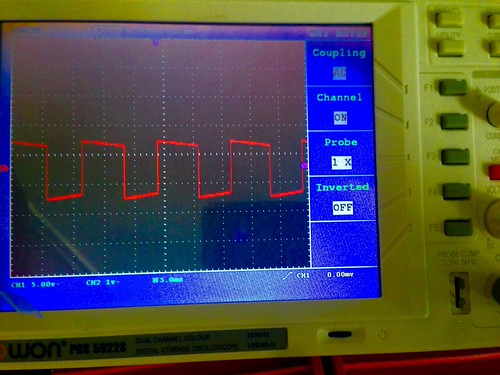Straight out of the MITx 6.002x class. We were talking about Op Amps and how to combine positive and negative feedback to create a voltage oscillation (capacitor charge/discharge). Of course I could only think about synthesizers, which as you’ll see is exactly where I took this. But first, the nitty-gritty.

The circuit layout:On the inverting side of the Op Amp, there’s a simple RC circuit. On the non-inverting side, there’s a positive feedback loop. This creates hysteresis, which ensures that its voltage there,$v^{+}$, remains at either +/- the voltage divider ratio,$\frac{V_1}{2}$. As the capacitor begins charging through the top resistor, the voltage difference$v^{+} - v^{-}$ will eventually flip signs causing the positive voltage to switch signs. There it will stay (via hysteresis) as the capacitor discharges until the difference becomes positive, and so on creating an oscillation on the values of the output from 4.5V to -4.5V and back.

You can determine the frequency of these oscillations using a mix of the node voltage method and the decay formulas for capacitors (an ODE). Doing so, yields the following:

T = 1/ =$2R_3C_1ln(1+\frac{2R_1}{R_2})$

So for equal sized resistors on the positive feedback side, this can be reduced to:
=$2R_3C_1ln(3)$

From there,  isolate the time constant,$RC$ as$RC$$\frac{1}{2fln(3)}$

The next trick is picking values of R and C that correspond to available sizes.

Starting with a 10K value of$R_3$ and aiming for a frequency of 440Hz (A440), gives a C value of ~1.034e-07, which in microfarads is ~0.103437. This means grab a .1 uF capacitor and you’re ready to go!

So here’s how it worked out (note: I replaced the 10K resistor with a 10K potentiometer):

The oscilloscope confirmed what I was hearing too:In other words, a square wave oscillator! You know, just like that cheese ball solo on Heart’s “Magic Man”

Yay circuits.

A note about the dual polarity voltage for Op Amps. I’m using a 741, which needs “positive” and “negative” voltage on ports 7 and 4. Incidentally what it really cares about is the relative difference. So 9, 4.5, 0 will work just the same as 4.5, 0, and -4.5. But here I used a voltage divider trick to split the voltage into a +/- side (check out this link: Instructables.com).Note that once you connect a load to your voltage source, it will change the resistance and thus throw your split into imbalance. To remedy this, use SMALL resistor values in the divider circuit (the previous link). I used 1K resistors. The reason this works is that the split is parallel, and thus, the smaller R value will mean less impact from a large load resistance. With 1K there was only a slight imbalance. At 10K it was much worse. A 1M, it was essentially useless.